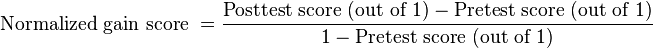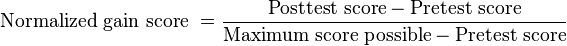# Normalized gain score$\mbox{Normalized gain score } = \frac{\mbox{Posttest score (out of 1)} - \mbox{Pretest score (out of 1)}}{1 - \mbox{Pretest score (out of 1)}}$$\mbox{Normalized gain score } = \frac{\mbox{Posttest score} - \mbox{Pretest score}}{\mbox{Maximum score possible} - \mbox{Pretest score}}$